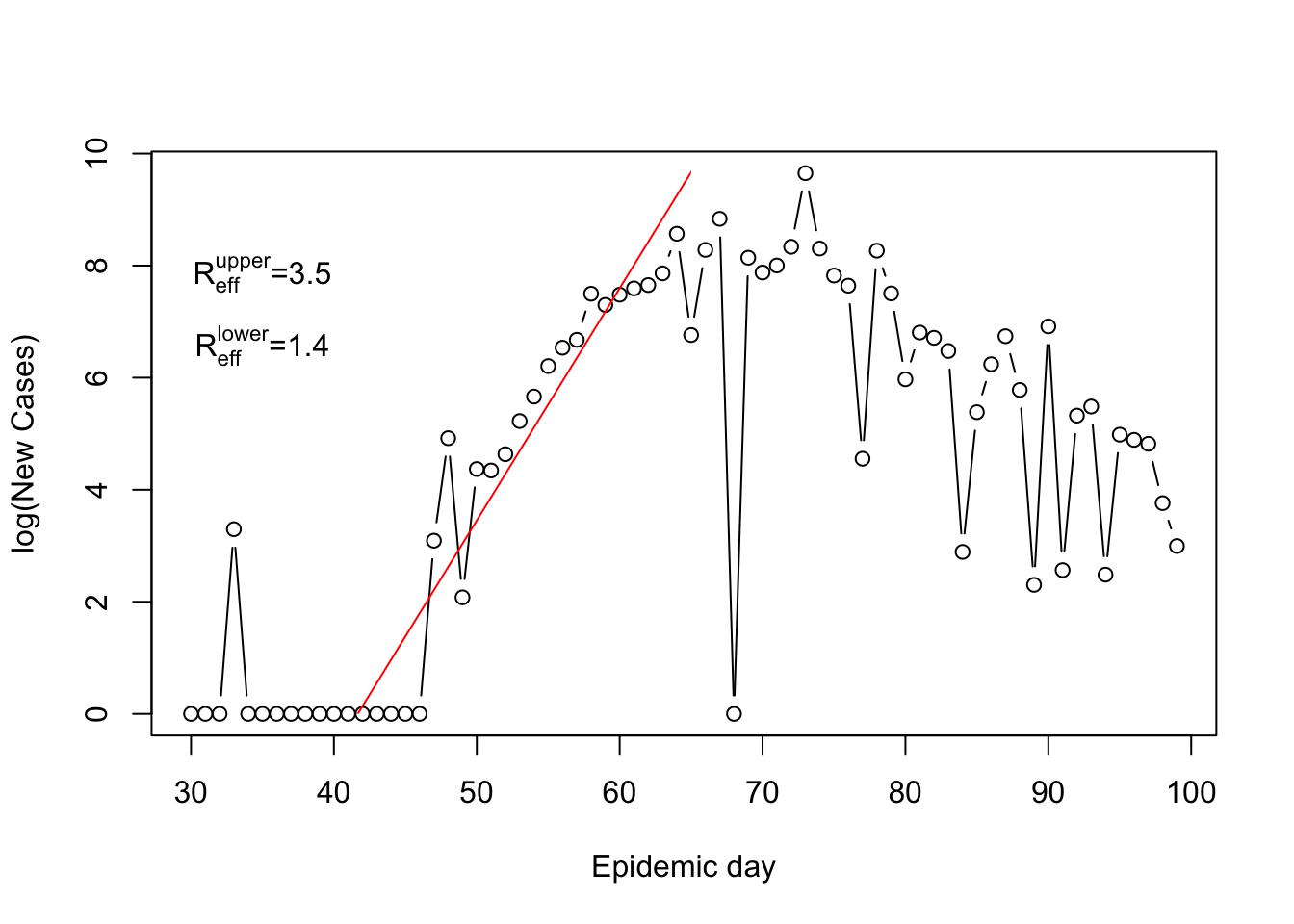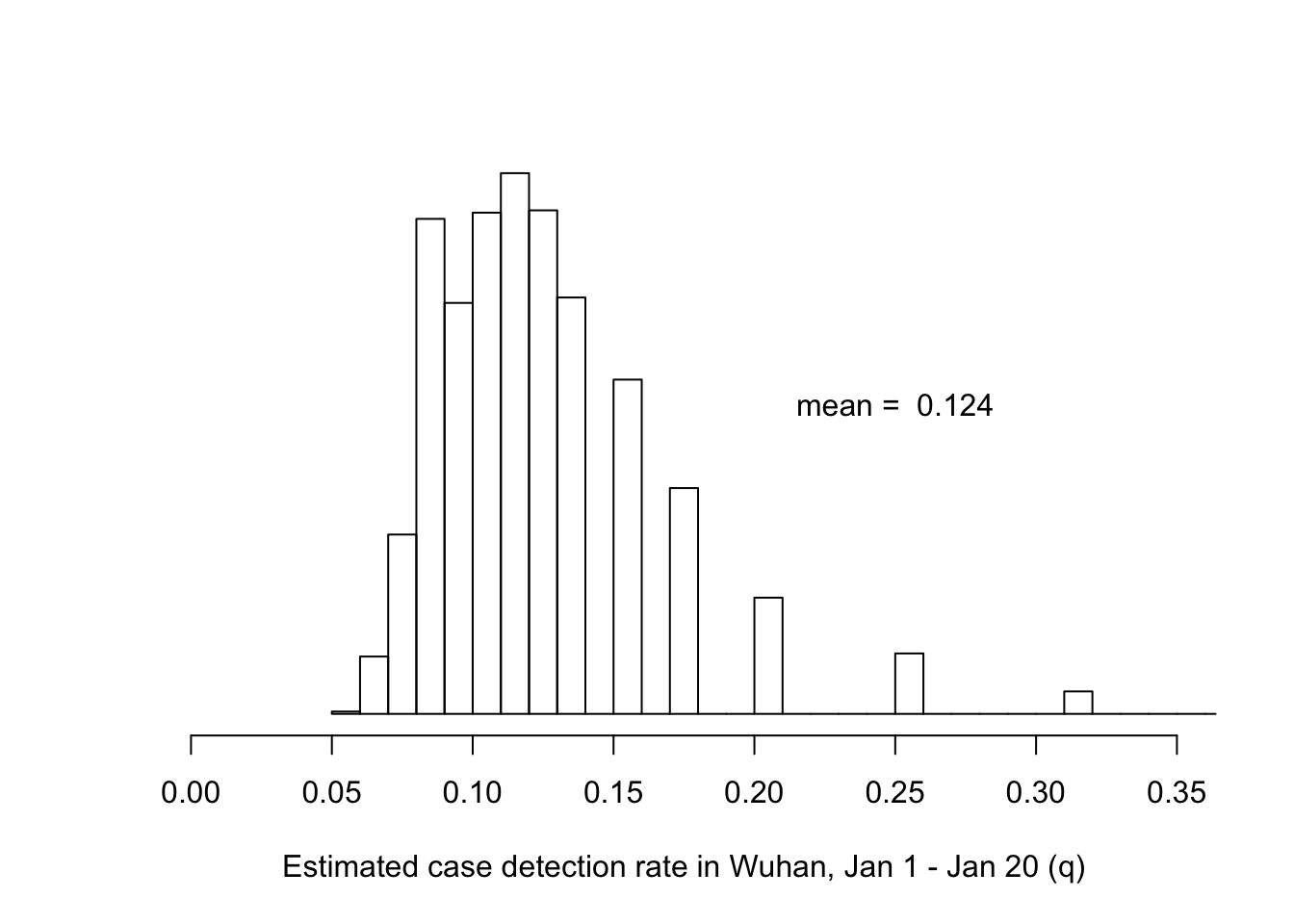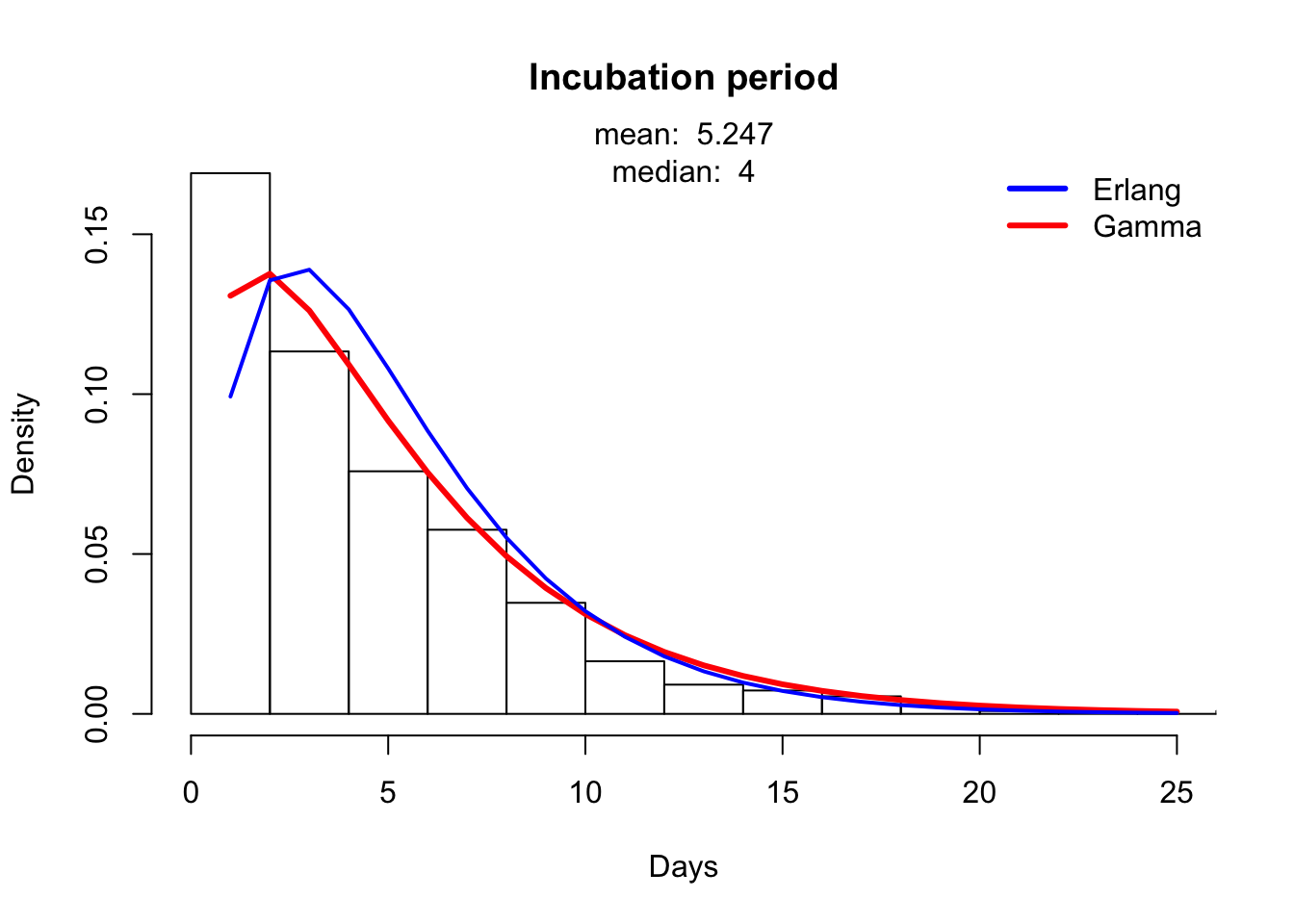### Basic reproduction number $$R_0$$

#### Overview

$$R_0$$, the basic reproduction number, is defined as the average number of secondary cases expected to arise from a single infected individual in a wholly susceptible population. $$R_{eff}$$, the effective reproduction number, refers to the expected number of secondary cases to arise from an arbitrary case at any point in time. $$R_{eff}$$ is expected to change over the course of an outbreak. Containment will occur when $$R_{eff}<1$$.

Estimating $$R_0$$ and $$R_{eff}$$ in this outbreak are challenging because: 1. There is little information from the first few infection generations 2. The distribution of incubation period and time from presentation of symptoms to hospitalization are not exponentially distributed 3. Interventions and policies intended to curtail the outbreak have affected the unfolding process and are therefore reflected in the case notification data.

#### Takeoff estimators

We have considered ‘’takeoff’’ estimators (e.g. Wearing et al. https://journals.plos.org/plosmedicine/article?id=10.1371/journal.pmed.0020174). Wearing et al. show that dynamics of an epidemic in the early phases are related to $$R_0$$. A regression line through plot of case notifications over time, from the start of the outbreak on 1 December 2020 (neglecting the first couple points), clearly does not go through the origin, suggesting that the epidemic was already in its exponential phase by Day 47 (Jan 16). If $$\gamma$$ were constant during this period after Day 47 (which it’s probably not, due to increasing case isolation), the number of cases would be expected to grow according to the proportionality

$\begin{equation} log(X_t) \propto (R_0-1)\gamma t. \end{equation}$

Although $$\gamma$$ is changing, we can nevertheless use this method to provide upper and lower bounds on $$R_{eff}$$ by looking at the plausible range of $$\gamma$$ (i.e., it’s minimum at $$\gamma \approx 1/7$$ and its maximum at $$\gamma \approx 1$$.Upper and lower bounds on $$R_0$$ for China using Wearing et al. method

A second approach looks at the first significant report in these data (41 cumulative cases on Day 33, Jan 3). During the pre-exponential phase of the epidemic, the takeoff rate is given by

$\begin{equation} R_0 = \lambda_2(\lambda_2/(\sigma m)+1)^m/(\gamma (1-(\lambda_2/(\gamma n)+1)^{-n})), \end{equation}$

where $$\lambda_2$$ is the slope of the takeoff at the beginning of the epidemic, $$1/\sigma$$ is the average latent period, $$1/\gamma$$ is the average infectious period and $$m$$ and $$n$$ are the parameters of Erlang distributions for the interval (Wearing and Rohani).

For assumed initial case on Day $$t_1=1$$ and $$x=41$$ cases on Day $$t=33$$, we have $$\lambda_2 \approx \frac{\log x}{t-t_1} = \frac{\log 41}{33-1} = 0.11$$. Inserting into the formula yields the estimate:

Inserting into the formula yields the estimate: $$\hat R_0 \approx 2.28$$.

### Case detection rate ($$q$$)

We have sought to estimate the case detection rate by adjusting the case fatality rate for cases reported in Wuhan from January 1 - January 19 according to the presumed actual case fatality rate of 3% as the difference between these two estimates is primarily due to the under-reporting of less severe cases. For a derivation of our estimator, see https://github.com/CEIDatUGA/ncov-parameters. The following plot shows the estimated probability distribution of $$q$$.Probability distribution of case detection rate in Wuhan (January 1, 2020 - January 19, 2020).

### Incubation period ($$1/\sigma$$)

Travelers who acquired the infection in a location where transmission was occurring and developed symptoms at a later time provide an opportunity to estimate the incubation period. Using data from the Be Outbreak Prepared (BOP) linelist, we estimate the incubation period to have a mean of 5.25 days. By maximum likelihood, we have determined that $$k=2$$ is the most likely shape parameter for an Erlang distributed onset to isolation interval.Estimated and observed distribution of incubation times.

### Lag between symptom onset and isolation

A key determinant of transmission is the lag between the onset of symptoms and isolation. The inverse of this quantity is the isolation rate. By maximum likelihood, we have determined that $$k=2$$ is the most likely shape parameter for an Erlang distributed onset to hospitalization interval. We determined that $$k=3$$ is the most likley shape parameter for an Erlang distributed onset to confirmation interval.